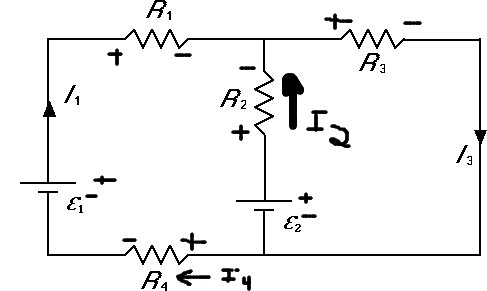Simple Circuit with Resistors and Two Batteries

Homework StatementThe circuit in the figure is composed of two batters (E1 = 5 V and E2 = 9 V) and four resistors (R1 = 110 W, R2 = 40 W, R3 = 50 W, and R4 = 50 W) as shown.

Find I1 and I3

Homework Equations

Well we need to apply KCL. I know the respective voltages (Ohm's law) need to add up to 0.

The Attempt at a Solution

I have tried like a million different equations for the 2 circuits present. I dont really understand circuits too well (which explains as to why I am posting/getting the wrong answer).

For the left loop:

I1R1 - I2R2 + E2 + I3R4 - E1 = 0 (Is it right to assume that I4 has equal current to I3?)

For the left loop:

I2R2 + I3R3 - E2 = 0

I1 + I2 = I3

To see the work that I did on the image to get the directions of the currents go to: http://i30.photobucket.com/albums/c309/hydr/showmepl-1.gif

I know the above equations are wrong, but I dont know whats wrong with them. They look right to me.

I solved for I1 first, but got the wrong answer. I know that if i add the left and right loop together then I should get the equation for the whole loop minus I2R2 and E2. I dont understand what Im doing wrong. I have inputed the respective values into a 3 x 3 matrix and solved for I1-3 with matlab, so there is no error in substitution.

Defennder
Homework Helper
I1R1 - I2R2 + E2 + I3R4 - E1 = 0 (Is it right to assume that I4 has equal current to I3?
There's your mistake. Where is I4 flowing? Where is I3? Is there any node (circuit junction) in between the points were I3 and I4 are flowing?

There's your mistake. Where is I4 flowing? Where is I3? Is there any node (circuit junction) in between the points were I3 and I4 are flowing?

So in other words, you're saying that since there is no potential difference between the negative sides of the batteries (where I4 is) then there is no current? Im sorry, im really confused with the current flow thing.

Defennder
Homework Helper
No, I'm not saying that. What is Kirchoff's current law here? How would you apply it to get I4? Do you even need to label the current separately as I4? Is it not already given by the expressions for the other current labels?

LowlyPion
Homework Helper
So in other words, you're saying that since there is no potential difference between the negative sides of the batteries (where I4 is) then there is no current? Im sorry, im really confused with the current flow thing.

Whoa. Wait a minute here. I4 must equal I1. Current in = current out of the battery.

You have to solve for I1 and I3.

I3 is only part of the loop equation for the 5V battery side as it affects R2

Whoa. Wait a minute here. I4 must equal I1. Current in = current out of the battery.

You have to solve for I1 and I3.

I3 is only part of the loop equation for the 5V battery side as it affects R2

Ok. Looking at the KCL, I understand, now, how I1 = I4. I also mislabeled my I2 current, it appears it should be facing south instead of north. Using this information and correcting my mistake relating the currents. I now have:

I1R1 + I2R2 + E2 + I1R4 - E1 = 0

-I2R2 + I3R3 - E2 = 0

I2 + I3 = I1

Am I correct to think that the I2 was initially incorrect?

Defennder
Homework Helper
It doesn't matter which direction you label your I2 current. It'll all work out negative if it's in the wrong direction. Your current equations appear correct, but your only mistake has got to do with I4 and nothing else.

It doesn't matter which direction you label your I2 current. It'll all work out negative if it's in the wrong direction. Your current equations appear correct, but your only mistake has got to do with I4 and nothing else.

Ok thanks a bunch. Im pretty sure I understand this now.